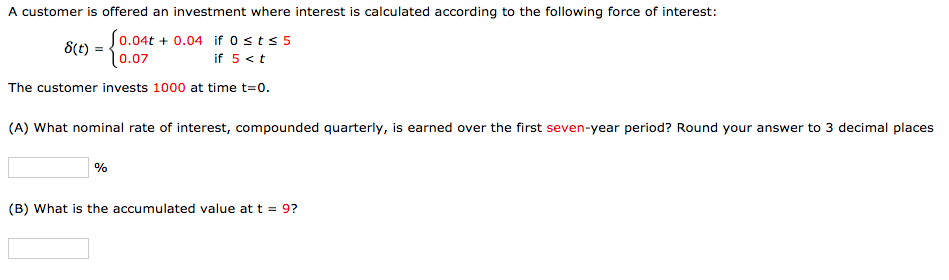Home / Answered Questions / Other / a-customer-is-offered-an-investment-where-interest-is-calculated-according-to-the-following-force-of-aw801

# (Solved): A Customer Is Offered An Investment Where Interest Is Calculated According To The Following Force Of...A customer is offered an investment where interest is calculated according to the following force of interest: 8(t) = 0.07 0.04t + 0.04 if Osts 5 10.07 if 5<t The customer invests 1000 at time t=0. (A) What nominal rate of interest, compounded quarterly, is earned over the first seven-year period? Round your answer to 3 decimal places % (B) What is the accumulated value at t = 9?

We have an Answer from Expert